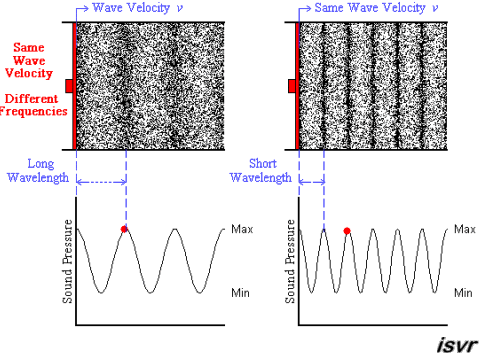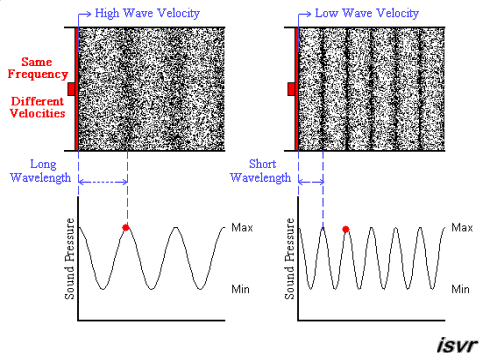Animations of Acoustic Waves
 WAVE BASICS Wavelength-Frequency Relation Let us now imagine one of those particles that moves back and forth as the high and low pressure peaks of the wave pass. The greater the rate at which the point moves back and forth, the greater the frequency (f) of the wave (defined as the number of oscillation cycles a particle makes each second, and given the units of Hertz, Hz, which has dimensions of (1/time)). It is the inverse of the period (in seconds) which measures the amount of time it takes for a wave to complete one oscillation or cycle. More generally, the distance between similar points on successive waves is called the wavelength λ (in metres). The speed at which the wave goes from one place to the other is called the wave velocity v (in metres per second). Frequency, wavelength and wave velocity are mathematically related by the equation:         The velocity of a  wave in a given medium (air, water, etc) is fixed and is related to the physical characteristics (temperature, density, etc...) of the medium. Frequency and wavelength, therefore, are inversely proportional to each other, i.e. higher frequencies correspond to shorter wavelengths. Moreover, this relation holds for any kind of wave phenomenon.   The animation below shows two acoustic longitudinal waves with two different frequencies but travelling with the same velocity. It can be seen that the wavelength is halved when the frequency is doubled.The next animation shows two acoustic longitudinal waves with the same frequency but travelling with two different velocities. You can see that the wavelength is halved when the velocity is halved.